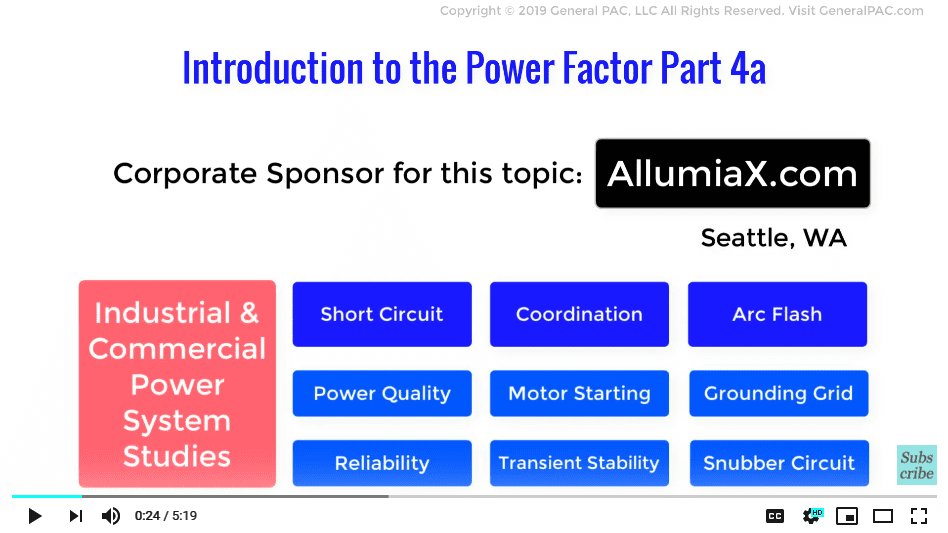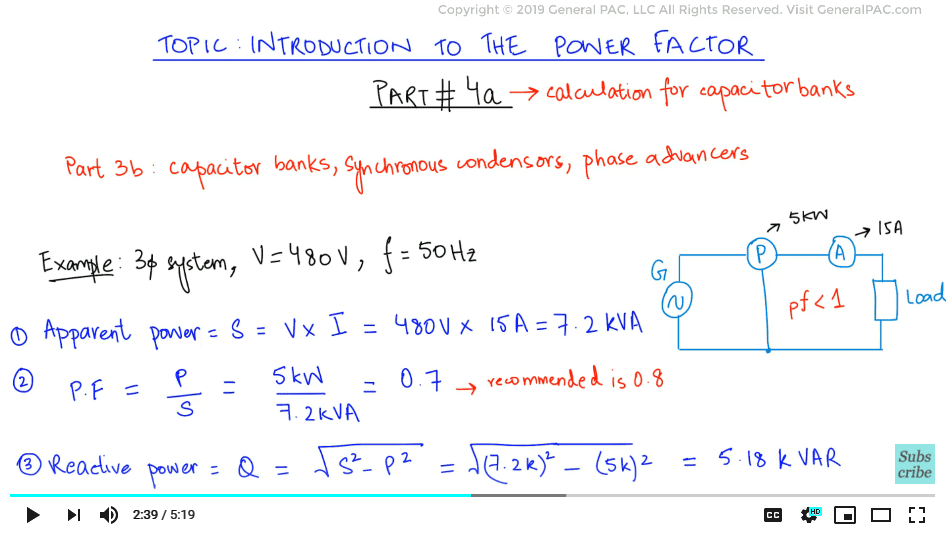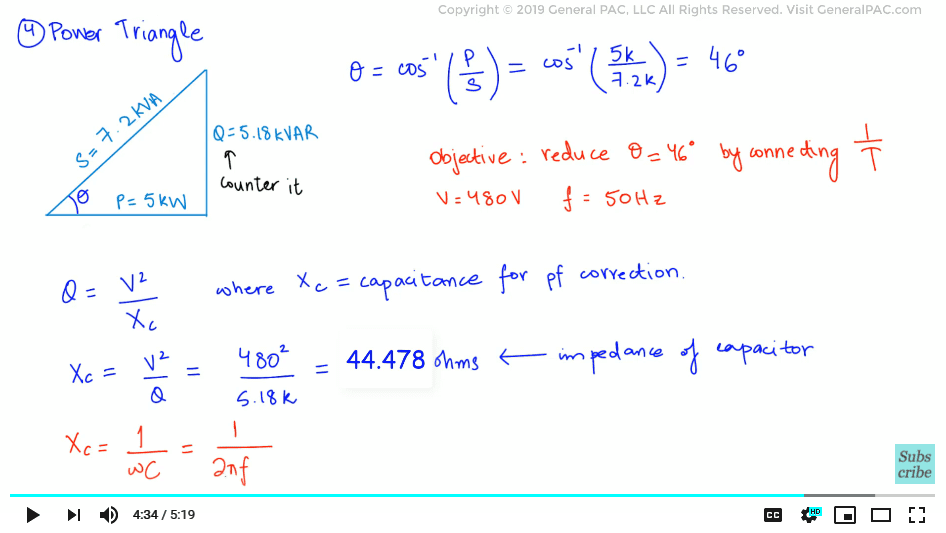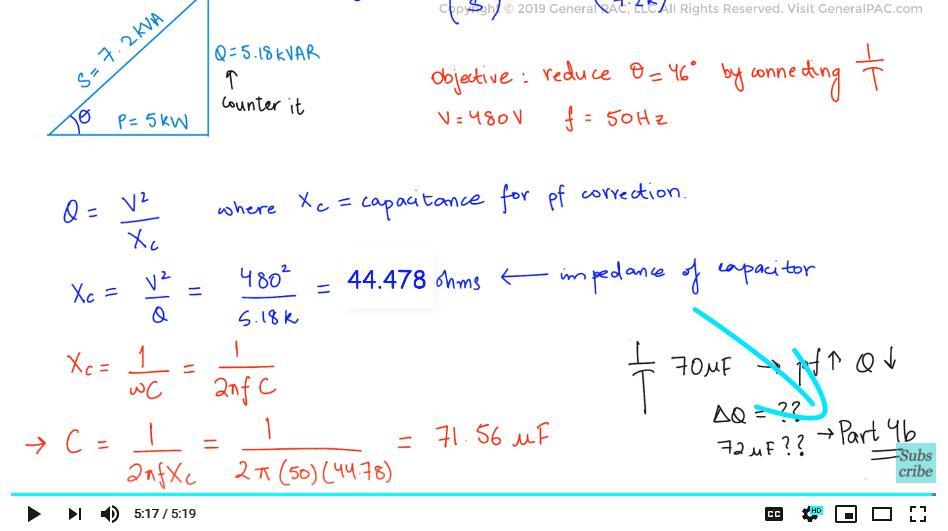This video was brought to you by GeneralPAC.com, making power systems Intuitive, Open and Free for Everyone, Everywhere. Consider subscribing and supporting through patreon.com/GeneralPAC. This is a mechanism for you to support us financially so we can continue making high quality power system video tutorials. Our corporate sponsor for this topic is AllumiaX.com from Seattle, Washington. Contact them for industrial and commercial power system studies.In the previous part, we looked at some of the equipment and techniques which we can use to improve the power factor of the system. In this part, we will be solving a practical example which will employ a capacitor for power factor correction.

Let’s draw a simple example consisting of a generator supplying an inductive load. We will be connecting an ammeter and wattmeter to measure the amount of real power and the current flowing in the system.It is a 3 phase system which is running at 480 volts with an operating frequency of 50Hz – instead of the 60 Hz frequency which is common in North America and other parts of the world. Now, say that the wattmeter reading shows a real power of 5kW and the ammeter shows a current reading of 15A. We will first calculate the apparent power of the system by multiplying the magnitudes of the voltage and current of the system.

Apparent power = V*I

Apparent power = (480 V) x (15A)

Apparent power = 7.2 kVA

So the apparent power is calculated at 7.2 kilo volt amperes. The difference between the magnitudes of the apparent and real power of the system shows the quality of the power factor, which suffice to say, is less than 1.

The greater the difference, the lower the quality of the power factor. If we want to calculate the existing power factor of the system, then we will use the equation established from previous parts as follows:

Power factor = Real Power/ Reactive power

Power factor = 5 kW/ 7.2 kVA

Power factor = 0.7

So the existing power factor comes out to be 0.7. In order to minimize system losses, a 0.8 or more power factor is generally recommended.

Since we now know the apparent power and the real power, we can calculate the reactive power using Pythagoras theorem.

Reactive power= √(apparent power)2 - (real power)2

Solving the theorem, we get a reactive power of 5.18 kilo VARS. The final preliminary step before analysis is to calculate the power factor angle. The next step is to analyze the system, and for that, we shall draw a power triangle.In order to find the phase angle between the apparent and real power, we will apply simple trigonometry to evaluate that the phase angle is the cosine inverse of the ratio between the apparent and real power. Therefore, we may calculate it as follows:

P.F = Cos-1(
5 KW / 702 KVA
)

The angle comes out to be approximately 46 degrees. Our objective is to connect a capacitor in parallel with the terminals of the inductive load in order to reduce this phase angle.

The first step of our analysis relates to the calculation of the size of the capacitor. Since we know the reactive power, we will need to select the proper size in order to counter its effects on the system. To achieve this, we will apply a derivative of the well-known Ohm’s law for reactive power as follows:

Reactive Power =
V2 / Xc

Where Xc is the capacitive reactance required for power factor correction. Rearranging the subject, we get the following equation

Xc =
V2 / Reactive Power
=
4802 / 5.18 KVAR

Plugging in the values and solving for X, we get a result of 44.478 ohms. This is the impedance of the capacitor which will be required to counteract the effects of the reactive power in the system.

The final step will involve the calculation of the value of capacitance. We know that the capacitance is inversely proportional to the product of angular frequency and the capacitive reactance. Using these relationships, we can derive the equation as follows:

Xc =
1 / wC
=
1 / fC

Plugging in the values for capacitive reactance and the operating frequency, we get a result of 71.56 microfarads. Using our engineering judgment, plugging in a capacitor with a size of 70 micro farads would drastically improve the power factor and reduce the reactive power. But by what magnitude? And on the other hand, why not opt for a capacitor of size 72 microfarads?

Let’s find out in part 4b.We hope, you have a continued interest in this topic and series as a student or professional. We also hope you find this content useful and enlightening. Please consider subscribing to GeneralPAC.com or becoming a patron on patreon.com.

GeneralPAC.com. Making Power Systems Intuitive and free to everyone, everywhere.

#### Greetings from the GeneralPAC Team!

We make high-quality Power Systems Video Tutorials on complex topics that are free and open to everyone!  Thank you so much for supporting us through Patreon so we can continue doing good and valuable work.

What is Patreon and why do we use it?

Patreon is a fantastic portal that allows our fans and community to make monthly contribution (like Netflix subscription) so we can continue creating high-quality power systems video tutorials. In return, you get access to incredible perks like voting on future topics, getting your questions answered, access to VIP Q/A webinars with the creators of GeneralPAC, and much more! We THANK YOU for supporting us

Why do we need your support?

An incredible amount of time and effort is needed to develop high-quality video tutorials. Each video (Part 1 for example) takes approximately 10 hours to complete which includes learning the concept ourselves, brainstorming creative ways to teach and explain the concepts, writing the script, audio recording, video recording, and editing. It's no wonder why Hundreds-of-Thousands of people have watched, liked, subscribed, and left positive comments on Youtube channel. Your support truly makes all the difference.